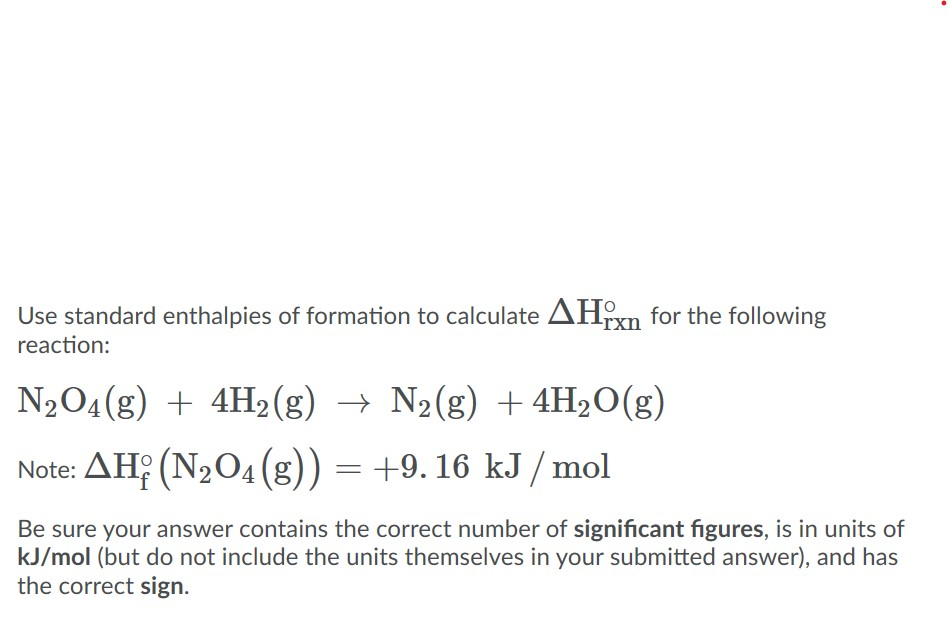# Use standard enthalpies of formation to calculate ΔH°rxn for the following reaction: N2O4 (g) + 4 H2 (g) → N2 (g) + 4 H2O (g) Note: ΔH°f (N2O4 (g) = + 9.16 kJ/mol. Be sure your answer contains the correct number of significant figures, is in units of kJ/mol (but do not include the units themselves in your submitted answer), and has the correct sign